/*! * \page Vector_7_sph_dlb Vector 7 SPH Dam break simulation with Dynamic load balacing * * * [TOC] * * * # SPH with Dynamic load Balancing # {#SPH_dlb} * * * This example show the classical SPH Dam break simulation with Load Balancing and Dynamic load balancing. With * Load balancing and Dynamic load balancing we indicate the possibility of the system to re-adapt the domain * decomposition to keep all the processor load and reduce idle time. * * \htmlonly * Simulation video 1
* * Simulation video 2
* * Simulation dynamic load balancing video 1
* * Simulation dynamic load balancing video 2
* * \endhtmlonly * * \htmlonly *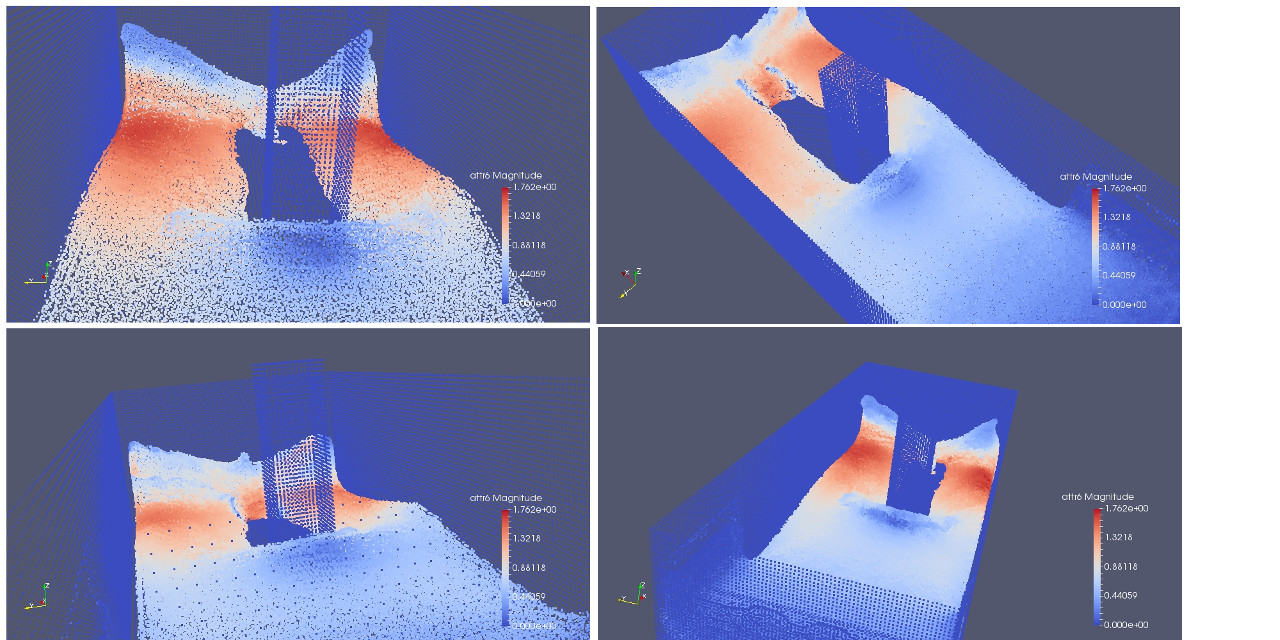* \endhtmlonly * * ## Inclusion ## {#e7_sph_inclusion} * * In order to use distributed vectors in our code we have to include the file Vector/vector_dist.hpp * we also include DrawParticles that has nice utilities to draw particles in parallel accordingly * to simple shapes * * \snippet Vector/7_SPH_dlb/main.cpp inclusion * */ //#define SE_CLASS1 //#define STOP_ON_ERROR //! \cond [inclusion] \endcond #include "Vector/vector_dist.hpp" #include #include "Draw/DrawParticles.hpp" //! \cond [inclusion] \endcond /*! * \page Vector_7_sph_dlb Vector 7 SPH Dam break simulation with Dynamic load balacing * * ## SPH simulation {#e7_sph_parameters} * * The SPH formulation used in this example code follow these equations * * \f$\frac{dv_a}{dt} = - \sum_{b = NN(a) } m_b \left(\frac{P_a + P_b}{\rho_a \rho_b} + \Pi_{ab} \right) \nabla_{a} W_{ab} + g \tag{1} \f$ * * \f$\frac{d\rho_a}{dt} = \sum_{b = NN(a) } m_b v_{ab} \cdot \nabla_{a} W_{ab} \tag{2} \f$ * * \f$P_a = b \left[ \left( \frac{\rho_a}{\rho_{0}} \right)^{\gamma} - 1 \right] \tag{3} \f$ * * with * * \f$\Pi_{ab} = \begin{cases} - \frac {\alpha \bar{c_{ab}} \mu_{ab} }{\bar{\rho_{ab}} } & v_{ab} \cdot r_{ab} > 0 \\ 0 & v_{ab} \cdot r_{ab} < 0 \end{cases} \tag{4}\f$ * * and the constants defined as * * \f$b = \frac{c_{s}^{2} \rho_0}{\gamma} \tag{5} \f$ * * \f$c_s = \sqrt{g \cdot h_{swl}} \tag{6} \f$ * * While the particle kernel support is given by * * \f$H = \sqrt{3 \cdot dp} \tag{7} \f$ * * Explain the equations is out of the context of this tutorial. An introduction * can be found regarding SPH in general in the original Monghagan SPH paper. * In this example we use the sligtly modified version * used by Dual-SPH (http://www.dual.sphysics.org/). A summary of the equation and constants can be founded in * their User Manual and the XML user Manual. * * ### Parameters {#e7_sph_parameters} * * Based on the equation * reported before several constants must be defined. * * \snippet Vector/7_SPH_dlb/main.cpp sim parameters * */ /*! \cond [sim parameters] \endcond */ // A constant to indicate boundary particles #define BOUNDARY 0 // A constant to indicate fluid particles #define FLUID 1 // initial spacing between particles dp in the formulas const double dp = 0.0085; // Maximum height of the fluid water // is going to be calculated and filled later on double h_swl = 0.0; // c_s in the formulas (constant used to calculate the sound speed) const double coeff_sound = 20.0; // gamma in the formulas const double gamma_ = 7.0; // sqrt(3.0*dp*dp) support of the kernel const double H = 0.0147224318643; // Eta in the formulas const double Eta2 = 0.01 * H*H; // alpha in the formula const double visco = 0.1; // cbar in the formula (calculated later) double cbar = 0.0; // Mass of the fluid particles const double MassFluid = 0.000614125; // Mass of the boundary particles const double MassBound = 0.000614125; // End simulation time const double t_end = 1.5; // Gravity acceleration const double gravity = 9.81; // Reference densitu 1000Kg/m^3 const double rho_zero = 1000.0; // Filled later require h_swl, it is b in the formulas double B = 0.0; // Constant used to define time integration const double CFLnumber = 0.2; // Minimum T const double DtMin = 0.00001; // Minimum Rho allowed const double RhoMin = 700.0; // Maximum Rho allowed const double RhoMax = 1300.0; // Filled in initialization double max_fluid_height = 0.0; // Properties // FLUID or BOUNDARY const size_t type = 0; // Density const int rho = 1; // Density at step n-1 const int rho_prev = 2; // Pressure const int Pressure = 3; // Delta rho calculated in the force calculation const int drho = 4; // calculated force const int force = 5; // velocity const int velocity = 6; // velocity at previous step const int velocity_prev = 7; /*! \cond [sim parameters] \endcond */ /*! \cond [vector_dist_def] \endcond */ // Type of the vector containing particles typedef vector_dist<3,double,aggregate> particles; // | | | | | | | | // | | | | | | | | // type density density Pressure delta force velocity velocity // at n-1 density at n - 1 /*! \cond [vector_dist_def] \endcond */ /*! \cond [model custom] \endcond */ struct ModelCustom { template inline void addComputation(Decomposition & dec, const vector & vd, size_t v, size_t p) { if (vd.template getProp(p) == FLUID) dec.addComputationCost(v,4); else dec.addComputationCost(v,3); } template inline void applyModel(Decomposition & dec, size_t v) { dec.setSubSubDomainComputationCost(v, dec.getSubSubDomainComputationCost(v) * dec.getSubSubDomainComputationCost(v)); } double distributionTol() { return 1.01; } }; /*! \cond [model custom] \endcond */ /*! * \page Vector_7_sph_dlb Vector 7 SPH Dam break simulation with Dynamic load balacing * * ### Equation of state {#e7_sph_equation_state} * * This function implement the formula 3 in the set of equations. It calculate the * pressure of each particle based on the local density of each particle. * * \snippet Vector/7_SPH_dlb/main.cpp eq_state_and_ker * */ /*! \cond [eq_state_and_ker] \endcond */ inline void EqState(particles & vd) { auto it = vd.getDomainIterator(); while (it.isNext()) { auto a = it.get(); double rho_a = vd.template getProp(a); double rho_frac = rho_a / rho_zero; vd.template getProp(a) = B*( rho_frac*rho_frac*rho_frac*rho_frac*rho_frac*rho_frac*rho_frac - 1.0); ++it; } } /*! \cond [eq_state_and_ker] \endcond */ /*! * \page Vector_7_sph_dlb Vector 7 SPH Dam break simulation with Dynamic load balancing * * ### Cubic SPH kernel and derivatives {#e7_sph_kernel} * * This function define the Cubic kernel or \f$W_{ab} \f$. The cubic kernel is * defined as * * \f$\begin{cases} 1.0 - \frac{3}{2} q^2 + \frac{3}{4} q^3 & 0 < q < 1 \\ (2 - q)^3 & 1 < q < 2 \\ 0 & q > 2 \end{cases} \f$ * * \snippet Vector/7_SPH_dlb/main.cpp kernel_sph * */ /*! \cond [kernel_sph] \endcond */ const double a2 = 1.0/M_PI/H/H/H; inline double Wab(double r) { r /= H; if (r < 1.0) return (1.0 - 3.0/2.0*r*r + 3.0/4.0*r*r*r)*a2; else if (r < 2.0) return (1.0/4.0*(2.0 - r*r)*(2.0 - r*r)*(2.0 - r*r))*a2; else return 0.0; } /*! \cond [kernel_sph] \endcond */ /*! * \page Vector_7_sph_dlb Vector 7 SPH Dam break simulation with Dynamic load balancing * * This function define the gradient of the Cubic kernel function \f$W_{ab} \f$. * * \f$\nabla W_{ab} = \beta (x,y,z) \f$ * * \f$\beta = \begin{cases} (c_1 q + d_1 q^2) & 0 < q < 1 \\ c_2 (2 - q)^2 & 1 < q < 2 \end{cases} \f$ * * \snippet Vector/7_SPH_dlb/main.cpp kernel_sph_der * */ /*! \cond [kernel_sph_der] \endcond */ const double c1 = -3.0/M_PI/H/H/H/H; const double d1 = 9.0/4.0/M_PI/H/H/H/H; const double c2 = -3.0/4.0/M_PI/H/H/H/H; const double a2_4 = 0.25*a2; // Filled later double W_dap = 0.0; inline void DWab(Point<3,double> & dx, Point<3,double> & DW, double r, bool print) { const double qq=r/H; if (qq < 1.0) { double qq2 = qq * qq; double fac = (c1*qq + d1*qq2)/r; DW.get(0) = fac*dx.get(0); DW.get(1) = fac*dx.get(1); DW.get(2) = fac*dx.get(2); } else if (qq < 2.0) { double wqq = (2.0 - qq); double fac = c2 * wqq * wqq / r; DW.get(0) = fac * dx.get(0); DW.get(1) = fac * dx.get(1); DW.get(2) = fac * dx.get(2); } else { DW.get(0) = 0.0; DW.get(1) = 0.0; DW.get(2) = 0.0; } } /*! \cond [kernel_sph_der] \endcond */ /*! * \page Vector_7_sph_dlb Vector 7 SPH Dam break simulation with Dynamic load balancing * * ### Tensile correction {#e7_sph_tensile} * * This function define the Tensile term. An explanation of the Tensile term is out of the * context of this tutorial, but in brief is an additional repulsive term that avoid the particles * to get too near. Can be considered at small scale like a repulsive force that avoid * particles to get too close like the Lennard-Jhonned potential at atomistic level. A good * reference is the Monaghan paper "SPH without a Tensile Instability" * * \snippet Vector/7_SPH_dlb/main.cpp tensile_term * * */ /*! \cond [tensile_term] \endcond */ // Tensile correction inline double Tensile(double r, double rhoa, double rhob, double prs1, double prs2) { const double qq=r/H; //-Cubic Spline kernel double wab; if(r>H) { double wqq1=2.0f-qq; double wqq2=wqq1*wqq1; wab=a2_4*(wqq2*wqq1); } else { double wqq2=qq*qq; double wqq3=wqq2*qq; wab=a2*(1.0f-1.5f*wqq2+0.75f*wqq3); } //-Tensile correction. double fab=wab*W_dap; fab*=fab; fab*=fab; //fab=fab^4 const double tensilp1=(prs1/(rhoa*rhoa))*(prs1>0? 0.01: -0.2); const double tensilp2=(prs2/(rhob*rhob))*(prs2>0? 0.01: -0.2); return (fab*(tensilp1+tensilp2)); } /*! \cond [tensile_term] \endcond */ /*! * * \page Vector_7_sph_dlb Vector 7 SPH Dam break simulation with Dynamic load balancing * * ### Viscous term {#e7_sph_viscous} * * This function implement the viscous term \f$\Pi_{ab} \f$ * * \snippet Vector/7_SPH_dlb/main.cpp viscous_term * * */ /*! \cond [viscous_term] \endcond */ inline double Pi(const Point<3,double> & dr, double rr2, Point<3,double> & dv, double rhoa, double rhob, double massb, double & visc) { const double dot = dr.get(0)*dv.get(0) + dr.get(1)*dv.get(1) + dr.get(2)*dv.get(2); const double dot_rr2 = dot/(rr2+Eta2); visc=std::max(dot_rr2,visc); if(dot < 0) { const float amubar=H*dot_rr2; const float robar=(rhoa+rhob)*0.5f; const float pi_visc=(-visco*cbar*amubar/robar); return pi_visc; } else return 0.0; } /*! \cond [viscous_term] \endcond */ /*! * * \page Vector_7_sph_dlb Vector 7 SPH Dam break simulation with Dynamic load balancing * * ### Force calculation {#e7_force_calc} * * Calculate forces. It calculate equation 1 and 2 in the set of formulas * * \snippet Vector/7_SPH_dlb/main.cpp calc_forces * * */ /*! \cond [calc_forces] \endcond */ template inline double calc_forces(particles & vd, CellList & NN, double & max_visc) { auto part = vd.getDomainIterator(); double visc = 0; // Update the cell-list vd.updateCellList(NN); // For each particle ... while (part.isNext()) { // ... a auto a = part.get(); // Get the position xp of the particle Point<3,double> xa = vd.getPos(a); // Take the mass of the particle dependently if it is FLUID or BOUNDARY double massa = (vd.getProp(a) == FLUID)?MassFluid:MassBound; // Get the density of the of the particle a double rhoa = vd.getProp(a); // Get the pressure of the particle a double Pa = vd.getProp(a); // Get the Velocity of the particle a Point<3,double> va = vd.getProp(a); // Reset the force counter (- gravity on zeta direction) vd.template getProp(a) = 0.0; vd.template getProp(a) = 0.0; vd.template getProp(a) = -gravity; vd.template getProp(a) = 0.0; // We threat FLUID particle differently from BOUNDARY PARTICLES ... if (vd.getProp(a) != FLUID) { // If it is a boundary particle calculate the delta rho based on equation 2 // This require to run across the neighborhoods particles of a auto Np = NN.template getNNIterator(NN.getCell(vd.getPos(a))); // For each neighborhood particle while (Np.isNext() == true) { // ... q auto b = Np.get(); // Get the position xp of the particle Point<3,double> xb = vd.getPos(b); // if (p == q) skip this particle if (a.getKey() == b) {++Np; continue;}; // get the mass of the particle double massb = (vd.getProp(b) == FLUID)?MassFluid:MassBound; // Get the velocity of the particle b Point<3,double> vb = vd.getProp(b); // Get the pressure and density of particle b double Pb = vd.getProp(b); double rhob = vd.getProp(b); // Get the distance between p and q Point<3,double> dr = xa - xb; // take the norm of this vector double r2 = norm2(dr); // If the particles interact ... if (r2 < 4.0*H*H) { // ... calculate delta rho double r = sqrt(r2); Point<3,double> dv = va - vb; Point<3,double> DW; DWab(dr,DW,r,false); const double dot = dr.get(0)*dv.get(0) + dr.get(1)*dv.get(1) + dr.get(2)*dv.get(2); const double dot_rr2 = dot/(r2+Eta2); max_visc=std::max(dot_rr2,max_visc); vd.getProp(a) += massb*(dv.get(0)*DW.get(0)+dv.get(1)*DW.get(1)+dv.get(2)*DW.get(2)); } ++Np; } } else { // If it is a fluid particle calculate based on equation 1 and 2 // Get an iterator over the neighborhood particles of p auto Np = NN.template getNNIterator(NN.getCell(vd.getPos(a))); // For each neighborhood particle while (Np.isNext() == true) { // ... q auto b = Np.get(); // Get the position xp of the particle Point<3,double> xb = vd.getPos(b); // if (p == q) skip this particle if (a.getKey() == b) {++Np; continue;}; double massb = (vd.getProp(b) == FLUID)?MassFluid:MassBound; Point<3,double> vb = vd.getProp(b); double Pb = vd.getProp(b); double rhob = vd.getProp(b); // Get the distance between p and q Point<3,double> dr = xa - xb; // take the norm of this vector double r2 = norm2(dr); // if they interact if (r2 < 4.0*H*H) { double r = sqrt(r2); Point<3,double> v_rel = va - vb; Point<3,double> DW; DWab(dr,DW,r,false); double factor = - massb*((vd.getProp(a) + vd.getProp(b)) / (rhoa * rhob) + Tensile(r,rhoa,rhob,Pa,Pb) + Pi(dr,r2,v_rel,rhoa,rhob,massb,visc)); vd.getProp(a) += factor * DW.get(0); vd.getProp(a) += factor * DW.get(1); vd.getProp(a) += factor * DW.get(2); vd.getProp(a) += massb*(v_rel.get(0)*DW.get(0)+v_rel.get(1)*DW.get(1)+v_rel.get(2)*DW.get(2)); } ++Np; } } ++part; } } /*! \cond [calc_forces] \endcond */ /*! * * \page Vector_7_sph_dlb Vector 7 SPH Dam break simulation with Dynamic load balancing * * ### Integration and dynamic time integration {#e7_delta_time_t} * * This function calculate the Maximum acceleration and velocity across the particles. * It is used to calculate a dynamic time-stepping. * * \snippet Vector/7_SPH_dlb/main.cpp max_acc_vel * * */ /*! \cond [max_acc_vel] \endcond */ void max_acceleration_and_velocity(particles & vd, double & max_acc, double & max_vel) { // Calculate the maximum acceleration auto part = vd.getDomainIterator(); while (part.isNext()) { auto a = part.get(); Point<3,double> acc(vd.getProp(a)); double acc2 = norm2(acc); Point<3,double> vel(vd.getProp(a)); double vel2 = norm2(vel); if (vel2 >= max_vel) max_vel = vel2; if (acc2 >= max_acc) max_acc = acc2; ++part; } max_acc = sqrt(max_acc); max_vel = sqrt(max_vel); Vcluster & v_cl = create_vcluster(); v_cl.max(max_acc); v_cl.max(max_vel); v_cl.execute(); } /*! \cond [max_acc_vel] \endcond */ /*! * * \page Vector_7_sph_dlb Vector 7 SPH Dam break simulation with Dynamic load balancing * * In this example we are using Dynamic time-stepping. The Dynamic time stepping is * calculated with the Courant-Friedrich-Lewy condition. See Monaghan 1992 "Smoothed Particle Hydrodynamic" * * \f$\delta t = CFL \cdot min(t_f,t_{cv}) \f$ * * where * * \f$\delta t_f = min \sqrt{h/f_a}\f$ * * \f$\delta t_{cv} = min \frac{h}{c_s + max \left| \frac{hv_{ab} \cdot r_{ab}}{r_{ab}^2} \right|} \f$ * * * \snippet Vector/7_SPH_dlb/main.cpp dyn_stepping * * */ /*! \cond [dyn_stepping] \endcond */ double calc_deltaT(particles & vd, double ViscDtMax) { double Maxacc = 0.0; double Maxvel = 0.0; max_acceleration_and_velocity(vd,Maxacc,Maxvel); //-dt1 depends on force per unit mass. const double dt_f = (Maxacc)?sqrt(H/Maxacc):std::numeric_limits::max(); //-dt2 combines the Courant and the viscous time-step controls. const double dt_cv = H/(std::max(cbar,Maxvel*10.) + H*ViscDtMax); //-dt new value of time step. double dt=double(CFLnumber)*std::min(dt_f,dt_cv); if(dt to_remove; size_t cnt = 0; void verlet_int(particles & vd, double dt) { // list of the particle to remove to_remove.clear(); // particle iterator auto part = vd.getDomainIterator(); double dt205 = dt*dt*0.5; double dt2 = dt*2.0; // For each particle ... while (part.isNext()) { // ... a auto a = part.get(); // if the particle is boundary if (vd.template getProp(a) == BOUNDARY) { // Update rho double rhop = vd.template getProp(a); // Update only the density vd.template getProp(a) = 0.0; vd.template getProp(a) = 0.0; vd.template getProp(a) = 0.0; vd.template getProp(a) = vd.template getProp(a) + dt2*vd.template getProp(a); vd.template getProp(a) = rhop; ++part; continue; } //-Calculate displacement and update position / Calcula desplazamiento y actualiza posicion. double dx = vd.template getProp(a)*dt + vd.template getProp(a)*dt205; double dy = vd.template getProp(a)*dt + vd.template getProp(a)*dt205; double dz = vd.template getProp(a)*dt + vd.template getProp(a)*dt205; vd.getPos(a) += dx; vd.getPos(a) += dy; vd.getPos(a) += dz; double velX = vd.template getProp(a); double velY = vd.template getProp(a); double velZ = vd.template getProp(a); double rhop = vd.template getProp(a); vd.template getProp(a) = vd.template getProp(a) + vd.template getProp(a)*dt2; vd.template getProp(a) = vd.template getProp(a) + vd.template getProp(a)*dt2; vd.template getProp(a) = vd.template getProp(a) + vd.template getProp(a)*dt2; vd.template getProp(a) = vd.template getProp(a) + dt2*vd.template getProp(a); // Check if the particle go out of range in space and in density if (vd.getPos(a) < 0.000263878 || vd.getPos(a) < 0.000263878 || vd.getPos(a) < 0.000263878 || vd.getPos(a) > 0.000263878+1.59947 || vd.getPos(a) > 0.000263878+0.672972 || vd.getPos(a) > 0.000263878+0.903944 || vd.template getProp(a) < RhoMin || vd.template getProp(a) > RhoMax) { to_remove.add(a.getKey()); } vd.template getProp(a) = velX; vd.template getProp(a) = velY; vd.template getProp(a) = velZ; vd.template getProp(a) = rhop; ++part; } // remove the particles vd.remove(to_remove,0); // increment the iteration counter cnt++; } void euler_int(particles & vd, double dt) { // list of the particle to remove to_remove.clear(); // particle iterator auto part = vd.getDomainIterator(); double dt205 = dt*dt*0.5; double dt2 = dt*2.0; // For each particle ... while (part.isNext()) { // ... a auto a = part.get(); // if the particle is boundary if (vd.template getProp(a) == BOUNDARY) { // Update rho double rhop = vd.template getProp(a); // Update only the density vd.template getProp(a) = 0.0; vd.template getProp(a) = 0.0; vd.template getProp(a) = 0.0; vd.template getProp(a) = vd.template getProp(a) + dt*vd.template getProp(a); vd.template getProp(a) = rhop; ++part; continue; } //-Calculate displacement and update position / Calcula desplazamiento y actualiza posicion. double dx = vd.template getProp(a)*dt + vd.template getProp(a)*dt205; double dy = vd.template getProp(a)*dt + vd.template getProp(a)*dt205; double dz = vd.template getProp(a)*dt + vd.template getProp(a)*dt205; vd.getPos(a) += dx; vd.getPos(a) += dy; vd.getPos(a) += dz; double velX = vd.template getProp(a); double velY = vd.template getProp(a); double velZ = vd.template getProp(a); double rhop = vd.template getProp(a); vd.template getProp(a) = vd.template getProp(a) + vd.template getProp(a)*dt; vd.template getProp(a) = vd.template getProp(a) + vd.template getProp(a)*dt; vd.template getProp(a) = vd.template getProp(a) + vd.template getProp(a)*dt; vd.template getProp(a) = vd.template getProp(a) + dt*vd.template getProp(a); // Check if the particle go out of range in space and in density if (vd.getPos(a) < 0.000263878 || vd.getPos(a) < 0.000263878 || vd.getPos(a) < 0.000263878 || vd.getPos(a) > 0.000263878+1.59947 || vd.getPos(a) > 0.000263878+0.672972 || vd.getPos(a) > 0.000263878+0.903944 || vd.template getProp(a) < RhoMin || vd.template getProp(a) > RhoMax) { to_remove.add(a.getKey()); } vd.template getProp(a) = velX; vd.template getProp(a) = velY; vd.template getProp(a) = velZ; vd.template getProp(a) = rhop; ++part; } // remove the particles vd.remove(to_remove,0); // increment the iteration counter cnt++; } /*! \cond [verlet_int] \endcond */ int main(int argc, char* argv[]) { /*! * * \page Vector_7_sph_dlb Vector 7 SPH Dam break simulation with Dynamic load balancing * * ## Main function {#e7_sph_main} * * Here we Initialize the library, we create a Box that define our domain, boundary conditions and ghost * * \see \ref e0_s_init * * \snippet Vector/7_SPH_dlb/main.cpp Initialization and parameters * */ //! \cond [Initialization and parameters] \endcond // initialize the library openfpm_init(&argc,&argv); // Here we define our domain a 2D box with internals from 0 to 1.0 for x and y Box<3,double> domain({-0.05,-0.05,-0.05},{1.7010,0.7065,0.5025}); size_t sz = {207,90,66}; // Fill W_dap W_dap = 1.0/Wab(H/1.5); // Here we define the boundary conditions of our problem size_t bc={NON_PERIODIC,NON_PERIODIC,NON_PERIODIC}; // extended boundary around the domain, and the processor domain Ghost<3,double> g(2*H); //! \cond [Initialization and parameters] \endcond /*! * \page Vector_7_sph_dlb Vector 7 SPH Dam break simulation with Dynamic load balancing * * ### Vector create {#e7_sph_vcreate} * * Here we define a distributed vector in 3D, we use the particles type that we defined previously. * Each particle contain the following properties * * **type** Type of the particle * * **rho** Density of the particle * * **rho_prev** Density at previous timestep * * **Pressure** Pressure of the particle * * **drho** Derivative of the density over time * * **force** acceleration of the particles * * **velocity** velocity of the particles * * **velocity_prev** velocity of the particles at previous time-step * * * In this case the vector contain 0 particles initially * * \see \ref e0_s_vector_inst * * The option DEC_GRAN(512) is related to the Load-Balancing decomposition * granularity. It indicate that the space must be decomposed by at least * * \f$N_{subsub} = 512 \cdot N_p \f$ * * Where \f$N_{subsub} \f$ is the number of sub-sub-domain in which the space * must be decomposed and \f$N_p \f$ is the number of processors. (The concept * of sub-sub-domain will be explained leter) * * \snippet Vector/7_SPH_dlb/main.cpp vector inst * \snippet Vector/7_SPH_dlb/main.cpp vector_dist_def * */ //! \cond [vector inst] \endcond particles vd(0,domain,bc,g,DEC_GRAN(512)); //! \cond [vector inst] \endcond /*! * \page Vector_7_sph_dlb Vector 7 SPH Dam break simulation with Dynamic load balancing * * ### Draw particles and initialization ## {#e7_sph_draw_part_init} * * In this part we initialize the problem creating particles. In order to do it we use the class DrawParticles. Because some of * the simulation constants require the maximum height \f$h_{swl} \f$ of the fluid to be calculated * and the maximum fluid height is determined at runtime, some of the constants just after we create the * fluid particles * * ### Draw Fluid ### {#e7_sph_draw_part_fluid} * * The Function DrawParticles::DrawBox return an iterator that can be used to create particle in a predefined * box (smaller than the simulation domain) with a predefined spacing. * We start drawing the fluid particles, the initial pressure is initialized accordingly to the * Hydrostatic pressure given by: * * \f$P = \rho_{0} g (h_{max} - z) \f$ * * Where \f$h_{max} \f$ is the maximum height of the fluid. * The density instead is given by the equation (3). Assuming \f$\rho \f$ constant to * \f$\rho_{0} \f$ in the Hydrostatic equation is a good approximation. Velocity is * initialized to zero. * * \see \ref e0_s_vector_inst * * \htmlonly *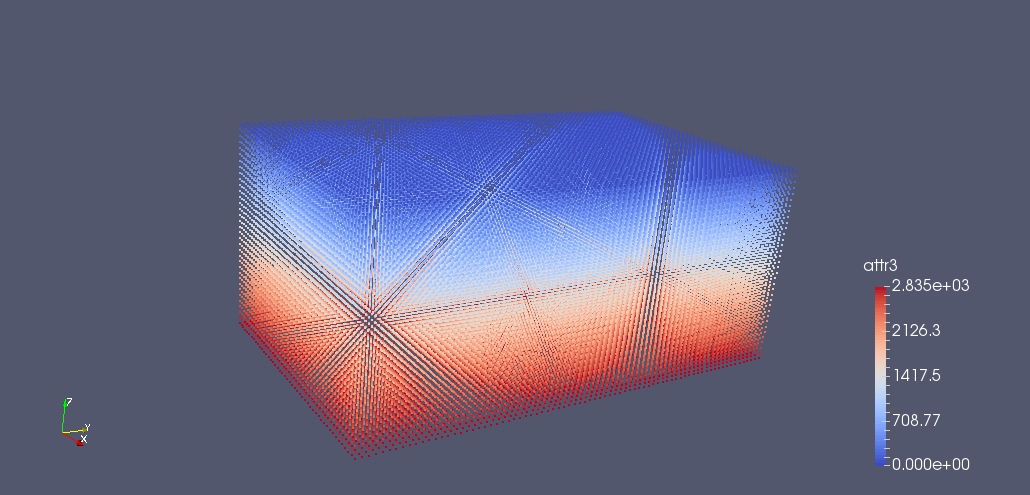* \endhtmlonly * * \snippet Vector/7_SPH_dlb/main.cpp draw fluid * */ //! \cond [draw fluid] \endcond // You can ignore all these dp/2.0 is a trick to reach the same initialization // of Dual-SPH that use a different criteria to draw particles Box<3,double> fluid_box({dp/2.0,dp/2.0,dp/2.0},{0.4+dp/2.0,0.67-dp/2.0,0.3+dp/2.0}); // return an iterator to the fluid particles to add to vd auto fluid_it = DrawParticles::DrawBox(vd,sz,domain,fluid_box); // here we fill some of the constants needed by the simulation max_fluid_height = fluid_it.getBoxMargins().getHigh(2); h_swl = fluid_it.getBoxMargins().getHigh(2) - fluid_it.getBoxMargins().getLow(2); B = (coeff_sound)*(coeff_sound)*gravity*h_swl*rho_zero / gamma_; cbar = coeff_sound * sqrt(gravity * h_swl); // for each particle inside the fluid box ... while (fluid_it.isNext()) { // ... add a particle ... vd.add(); // ... and set it position ... vd.getLastPos() = fluid_it.get().get(0); vd.getLastPos() = fluid_it.get().get(1); vd.getLastPos() = fluid_it.get().get(2); // and its type. vd.template getLastProp() = FLUID; // We also initialize the density of the particle and the hydro-static pressure given by // // rho_zero*g*h = P // // rho_p = (P/B + 1)^(1/Gamma) * rho_zero // vd.template getLastProp() = rho_zero * gravity * (max_fluid_height - fluid_it.get().get(2)); vd.template getLastProp() = pow(vd.template getLastProp() / B + 1, 1.0/gamma_) * rho_zero; vd.template getLastProp() = vd.template getLastProp(); vd.template getLastProp() = 0.0; vd.template getLastProp() = 0.0; vd.template getLastProp() = 0.0; vd.template getLastProp() = 0.0; vd.template getLastProp() = 0.0; vd.template getLastProp() = 0.0; // next fluid particle ++fluid_it; } //! \cond [draw fluid] \endcond /*! * \page Vector_7_sph_dlb Vector 7 SPH Dam break simulation with Dynamic load balancing * * ### Draw Recipient ### * * Here we draw the recipient using the function DrawParticles::DrawSkin. This function can draw a set * of particles inside a box A removed of a second box or an array of boxes. So all the particles in the * area included in the area A - B - C. There is no restriction that B or C must be included into A. * * \htmlonly *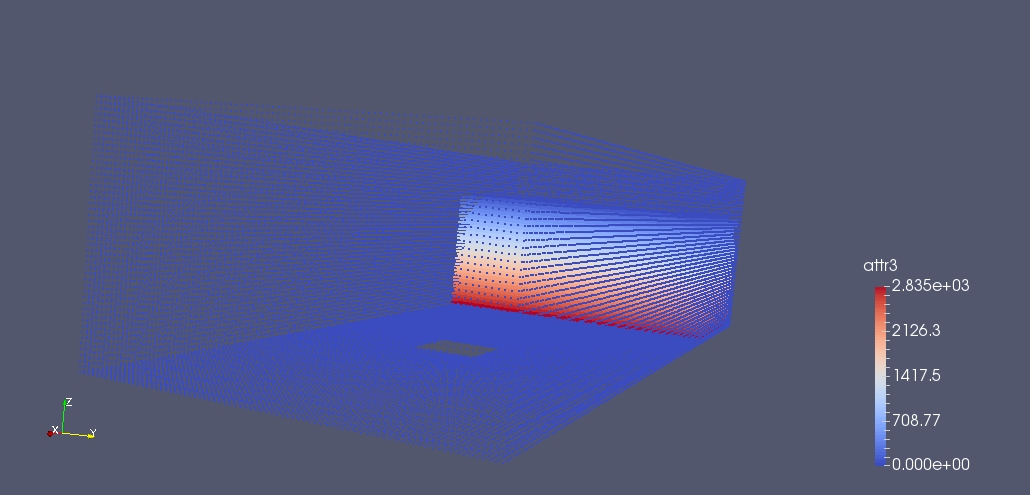* \endhtmlonly * * In this case A is the box defining the recipient, B is the box cutting out the internal * part of the recipient, C is the hole where we will place the obstacle. * Because we use Dynamic boundary condition (DBC) we initialize the density * to \f$\rho_{0} \f$. It will be update over time according to equation (3) to keep * the particles confined. * * \snippet Vector/7_SPH_dlb/main.cpp draw recipient * */ //! \cond [draw recipient] \endcond // Recipient Box<3,double> recipient1({0.0,0.0,0.0},{1.6+dp/2.0,0.67+dp/2.0,0.4+dp/2.0}); Box<3,double> recipient2({dp,dp,dp},{1.6-dp/2.0,0.67-dp/2.0,0.4+dp/2.0}); Box<3,double> obstacle1({0.9,0.24-dp/2.0,0.0},{1.02+dp/2.0,0.36,0.45+dp/2.0}); Box<3,double> obstacle2({0.9+dp,0.24+dp/2.0,0.0},{1.02-dp/2.0,0.36-dp,0.45-dp/2.0}); Box<3,double> obstacle3({0.9+dp,0.24,0.0},{1.02,0.36,0.45}); openfpm::vector> holes; holes.add(recipient2); holes.add(obstacle1); auto bound_box = DrawParticles::DrawSkin(vd,sz,domain,holes,recipient1); while (bound_box.isNext()) { vd.add(); vd.getLastPos() = bound_box.get().get(0); vd.getLastPos() = bound_box.get().get(1); vd.getLastPos() = bound_box.get().get(2); vd.template getLastProp() = BOUNDARY; vd.template getLastProp() = rho_zero; vd.template getLastProp() = rho_zero; vd.template getLastProp() = 0.0; vd.template getLastProp() = 0.0; vd.template getLastProp() = 0.0; vd.template getLastProp() = 0.0; vd.template getLastProp() = 0.0; vd.template getLastProp() = 0.0; ++bound_box; } //! \cond [draw recipient] \endcond /*! * \page Vector_7_sph_dlb Vector 7 SPH Dam break simulation with Dynamic load balancing * * ### Draw Obstacle ### * * Here we draw the obstacle in the same way we draw the recipient. also for the obstacle * is valid the same concept of using Dynamic boundary condition (DBC) * * \htmlonly *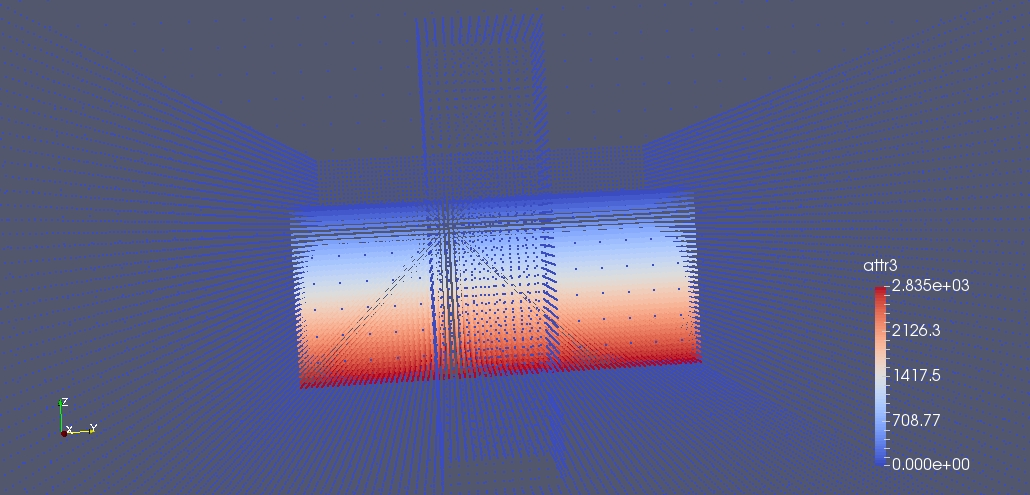* \endhtmlonly * * \snippet Vector/7_SPH_dlb/main.cpp draw obstacle * */ //! \cond [draw obstacle] \endcond auto obstacle_box = DrawParticles::DrawSkin(vd,sz,domain,obstacle2,obstacle1); while (obstacle_box.isNext()) { vd.add(); vd.getLastPos() = obstacle_box.get().get(0); vd.getLastPos() = obstacle_box.get().get(1); vd.getLastPos() = obstacle_box.get().get(2); vd.template getLastProp() = BOUNDARY; vd.template getLastProp() = rho_zero; vd.template getLastProp() = rho_zero; vd.template getLastProp() = 0.0; vd.template getLastProp() = 0.0; vd.template getLastProp() = 0.0; vd.template getLastProp() = 0.0; vd.template getLastProp() = 0.0; vd.template getLastProp() = 0.0; ++obstacle_box; } vd.map(); //! \cond [draw obstacle] \endcond /*! * \page Vector_7_sph_dlb Vector 7 SPH Dam break simulation with Dynamic load balancing * * ## Load balancing and Dynamic load balancing ## * * ### Load Balancing ### * * If at this point we output the particles and we visualize where they are accordingly * to their processor id we can easily see that particles are distributed unevenly. The * processor that has particles in white has few particles and all of them are non fluid. * This mean that it will be almost in idle. This situation is not ideal * * \htmlonly *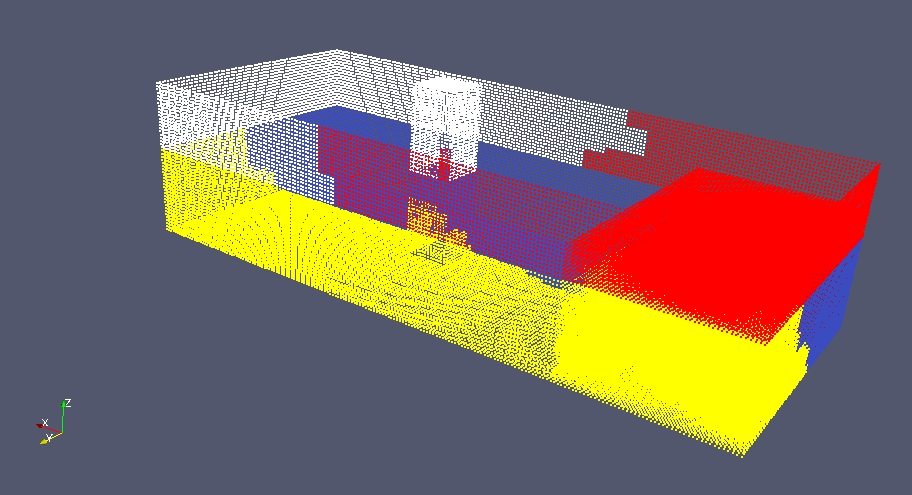* \endhtmlonly * * In order to reach an optimal situation we have to distribute the particles to * reach a balanced situation. To do this we have to set the computation of each * sub-sub-domain, redecompose the space and distribute the particles accordingly to this * new configuration. To do this we need a model. A model specify how to set * the computational cost for each sub-sub-domains (for example it specify if the computational cost to * process a sub-sub-domain is quadratic or linear with the number of * particles ...). A model look like this. * * \snippet Vector/7_SPH_dlb/main.cpp model custom * * Setting the the computational cost on sub-sub-domains is performed running * across the particles. For each one of them, it is calculated on which sub-sub-domain it belong. * Than the function **addComputation** is called. Inside this call we can set the weight * in the way we prefer. In this case we set the weight as: * * \f$w_v = 4 N_{fluid} + 3 N_{boundary} \f$ * * Where \f$N_{fluid} \f$ Is the number of fluid particles in the sub-sub-domains and \f$N_{boundary} \f$ * are the number of boundary particles. For example in our ModelCustom we square this number, * because the computation is proportional to the square of the number of particles in each sub-sub-domain. * A second cycle is performed in order to calculate a complex function of this number (for example squaring). * * Implicitly the communication cost is given by \f$\frac{V_{ghost}}{V_{sub-sub}} * t_s \f$, while the migration cost is given by \f$v_{sub-sub} \f$. In general\f$t_s \f$ is the number * of ghost get between two rebalance. In this special case where we have two type of particles, * we have two different computation for each of them, this mean that fluid particles * and boundary particles has different computation cost. * * After filling the computational cost based on our model * we can decompose the problem in computationally equal chunk for each processor. * We use the function **decomposed** to redecompose the space and subsequently we use * the function map to redistribute * the particles. * * \note All processors now has part of the fluid. It is good to note that the computationaly * balanced configuration does not correspond to the evenly distributed particles to know * more about that please follow the video tutorials * * \htmlonly * Dynamic load balancing the theory part1 * * Dynamic load balancing the theory part2 * * Dynamic load balancing practice part1 * * \endhtmlonly * * \snippet Vector/7_SPH_dlb/main.cpp load balancing * * \htmlonly *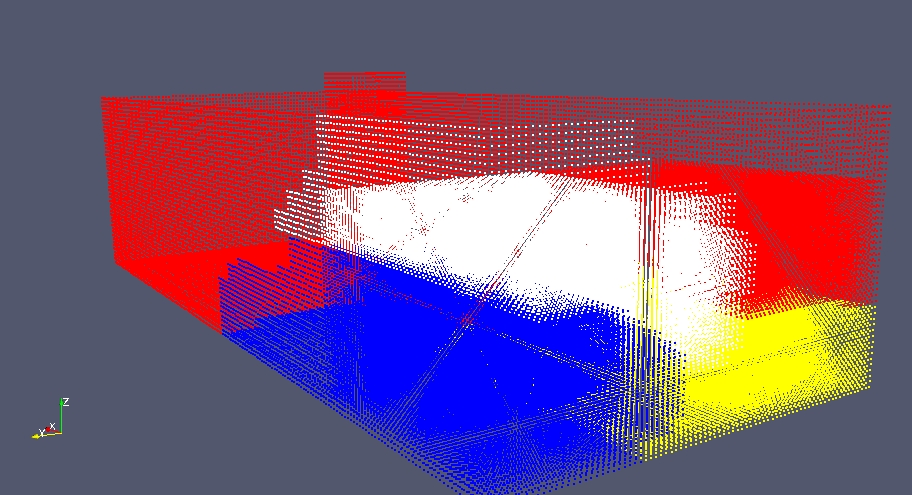* \endhtmlonly * */ //! \cond [load balancing] \endcond // Now that we fill the vector with particles ModelCustom md; vd.addComputationCosts(md); vd.getDecomposition().decompose(); vd.map(); //! \cond [load balancing] \endcond vd.ghost_get(); auto NN = vd.getCellList(2*H); // Evolve /*! * \page Vector_7_sph_dlb Vector 7 SPH Dam break simulation with Dynamic load balancing * * ## Main Loop ## * * The main loop do time integration. It calculate the pressure based on the * density, than calculate the forces, than we calculate delta time, and finally update position * and velocity. After 200 time-step we do a rebalancing. And we save the configuration * every 0.01 seconds * * \snippet Vector/7_SPH_dlb/main.cpp main loop * */ //! \cond [main loop] \endcond size_t write = 0; size_t it = 0; size_t it_reb = 0; double t = 0.0; while (t <= t_end) { Vcluster & v_cl = create_vcluster(); timer it_time; ////// Do rebalancing every 200 timesteps it_reb++; if (it_reb == 200) { vd.map(); it_reb = 0; ModelCustom md; vd.addComputationCosts(md); vd.getDecomposition().decompose(); if (v_cl.getProcessUnitID() == 0) std::cout << "REBALANCED " << std::endl; } vd.map(); // Calculate pressure from the density EqState(vd); double max_visc = 0.0; vd.ghost_get(); // Calc forces calc_forces(vd,NN,max_visc); // Get the maximum viscosity term across processors v_cl.max(max_visc); v_cl.execute(); // Calculate delta t integration double dt = calc_deltaT(vd,max_visc); // VerletStep or euler step it++; if (it < 40) verlet_int(vd,dt); else { euler_int(vd,dt); it = 0; } t += dt; if (write < t*100) { vd.write("Geometry",write); write++; if (v_cl.getProcessUnitID() == 0) std::cout << "TIME: " << t << " write " << it_time.getwct() << " " << v_cl.getProcessUnitID() << " " << cnt << std::endl; } else { if (v_cl.getProcessUnitID() == 0) std::cout << "TIME: " << t << " " << it_time.getwct() << " " << v_cl.getProcessUnitID() << " " << cnt << std::endl; } } //! \cond [main loop] \endcond /*! * * \page Vector_7_sph_dlb Vector 7 SPH Dam break simulation with Dynamic load balancing * * ## Finalize ## {#finalize_e0_sim} * * * At the very end of the program we have always de-initialize the library * * \snippet Vector/7_SPH_dlb/main.cpp finalize * */ //! \cond [finalize] \endcond openfpm_finalize(); //! \cond [finalize] \endcond /*! * \page Vector_7_sph_dlb Vector 7 SPH Dam break simulation with Dynamic load balancing * * ## Full code ## {#code_e7_sph_dlb} * * \include Vector/7_SPH_dlb/main.cpp * */ }